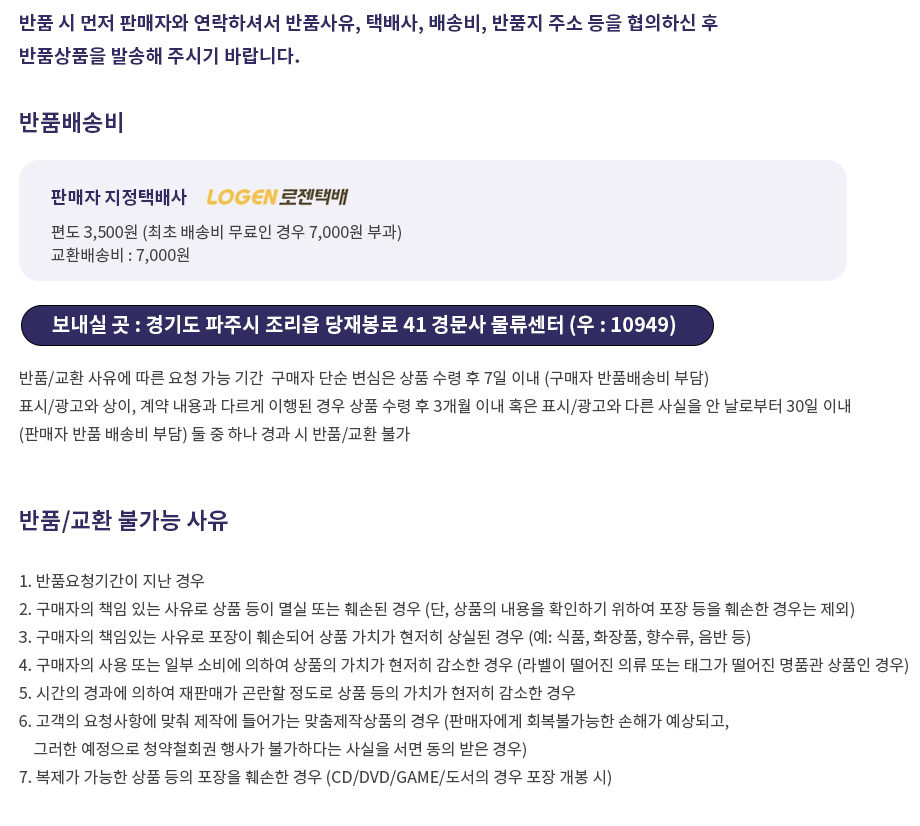## 로그인이필요합니다## 도서를 검색해 주세요.

원하시는 결과가 없으시면 문의 주시거나 다른 검색어를 입력해보세요.

•상품상세

## First Course in Differential Equations with Modeling Applications, 11th Metric Version 요약정보 및 구매

상품 선택옵션 0 개, 추가옵션 0 개

사용후기 0 개
지은이 Zill. Dennis G. 2017-04-20 11판 468 9781337556644 구매가능 47,000원 0점 주문시 결제

### 선택된 옵션

• First Course in Differential Equations with Modeling Applications, 11th Metric Version
+0원

## 관련상품

• First Course in Differential Equations with Modeling Applications, 11th Metric Version

• 1. INTRODUCTION TO DIFFERENTIAL EQUATIONS.

Definitions and Terminology. Initial-Value Problems. Differential Equations as Mathematical Models. Chapter 1 in Review.

2. FIRST-ORDER DIFFERENTIAL EQUATIONS.

Solution Curves Without a Solution. Separable Variables. Linear Equations. Exact Equations and Integrating Factors. Solutions by Substitutions. A Numerical Method. Chapter 2 in Review.

3. MODELING WITH FIRST-ORDER DIFFERENTIAL EQUATIONS.

Linear Models. Nonlinear Models. Modeling with Systems of First-Order Differential Equations. Chapter 3 in Review.

4. HIGHER-ORDER DIFFERENTIAL EQUATIONS.

Preliminary Theory-Linear Equations. Reduction of Order. Homogeneous Linear Equations with Constant Coefficients. Undetermined Coefficients-Superposition Approach. Undetermined Coefficients-Annihilator Approach. Variation of Parameters. Cauchy-Euler Equation. Solving Systems of Linear Differential Equations by Elimination. Nonlinear Differential Equations. Chapter 4 in Review.

5. MODELING WITH HIGHER-ORDER DIFFERENTIAL EQUATIONS.

Linear Models: Initial-Value Problems. Linear Models: Boundary-Value Problems. Nonlinear Models. Chapter 5 in Review.

6. SERIES SOLUTIONS OF LINEAR EQUATIONS.

Review of Power Series Solutions About Ordinary Points. Solutions About Singular Points. Special Functions. Chapter 6 in Review.

7. LAPLACE TRANSFORM.

Definition of the Laplace Transform. Inverse Transform and Transforms of Derivatives. Operational Properties I. Operational Properties II. Dirac Delta Function. Systems of Linear Differential Equations. Chapter 7 in Review.

8. SYSTEMS OF LINEAR FIRST-ORDER DIFFERENTIAL EQUATIONS.

Preliminary Theory. Homogeneous Linear Systems. Nonhomogeneous Linear Systems. Matrix Exponential. Chapter 8 in Review.

9. NUMERICAL SOLUTIONS OF ORDINARY DIFFERENTIAL EQUATIONS.

Euler Methods. Runge-Kutta Methods. Multistep Methods. Higher-Order Equations and Systems. Second-Order Boundary-Value Problems. Chapter 9 in Review.

Appendix I. Gamma Function.

Appendix II. Matrices.

Appendix III. Laplace Transforms.

• Dennis G. Zill is professor of mathematics at Loyola Marymount University. His interests are in applied mathematics, special functions, and integral transforms. Dr. Zill received his Ph.D. in applied mathematics and his M.S. from Iowa State University in 1967 and 1964, respectively. He received his B.A. from St. Mary's in Winona, Minnesota, in 1962. Dr. Zill also is former chair of the Mathematics Department at Loyola Marymount University. He is the author or co-author of 13 mathematics texts.

• 학습자료

등록된 학습자료가 없습니다.

정오표

등록된 정오표가 없습니다.

사용후기가 없습니다.

상품문의가 없습니다.

• ## 배송/교환정보

### 배송정보### 교환/반품 정보### 선택된 옵션

• First Course in Differential Equations with Modeling Applications, 11th Metric Version
+0원